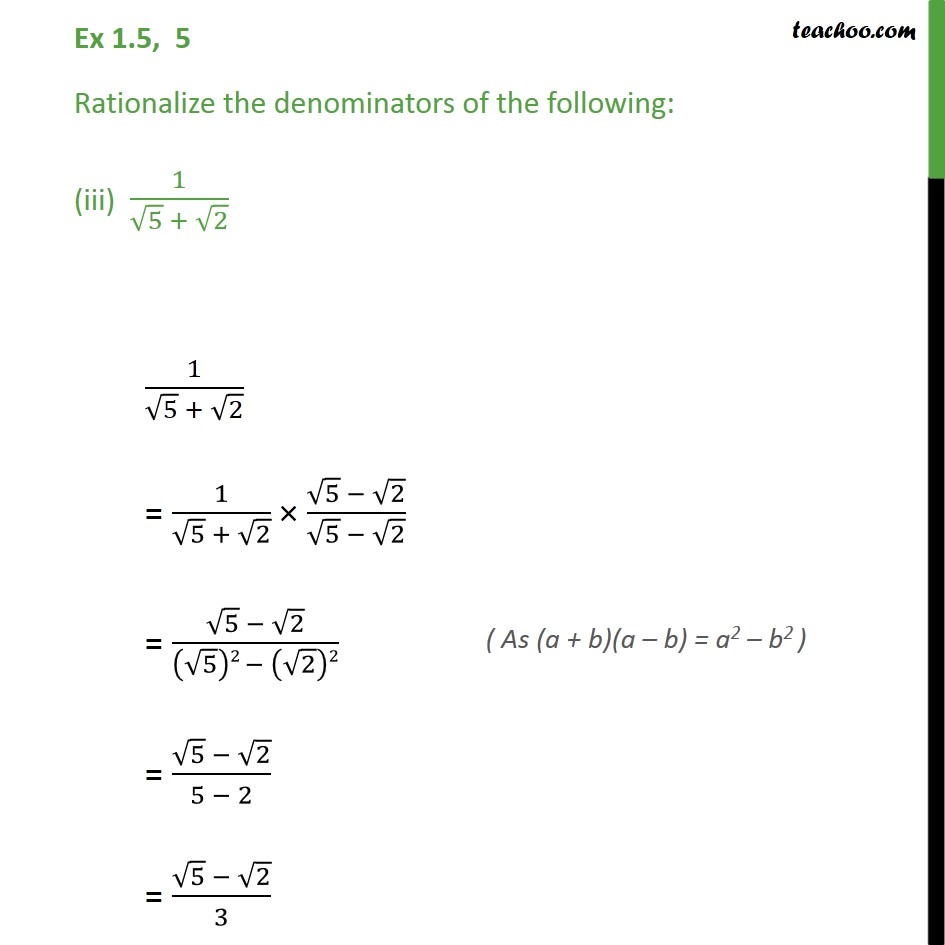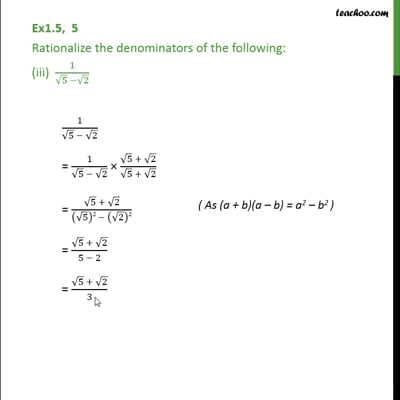Ex 1.5

Chapter 1 Class 9 Number Systems
Serial order wiseThis video is only available for Teachoo black users

Get live Maths 1-on-1 Classs - Class 6 to 12

### Transcript

Ex 1.5, 5 Rationalize the denominators of the following: (iii) 1/(√5 + √2) 1/(√5 + √2) = 1/(√5 + √2) × (√5 − √2)/(√5 − √2) = (√5 − √2)/((√5)2 − (√2)2) = (√5 − √2)/(5 − 2) = (√5 − √2)/3